Product Excellence Summit 2022: Oct 4th, 2022 — Online and in-person in San Francisco

# How we implemented SVG Arrows in React: The Curvature (2/3)

In the first part of this series, we made a very naive implementation of the component, where we drew a simple line between two points. Now, we will make a nicely curved curve from a straight line.

If you want to skip ahead, you can go directly to our code on CodeSandbox or Github.

## Cubic Bezier curve in SVG

To understand how to curve the line, we need to explain how Bezier curves are represented in SVG. We needed to make a cubic Bezier curve, which needs four points:

The Start point, where we are currently located in the SVG canvas, marks the beginning of the Bezier curve `[p1x, p1y]`. The other two points (`[p2x, p2y]` and `[p3x, p3y]`) indicate the Control points that determine the curvature, and the last point (`[p4x, p4y]`) indicates the End point of the curve. For the `path` component in SVG, the Bezier curve is marked with the letter `C`. For the `path` component, we would write it in the HTML pseudocode as follows (all `p1x...p4x`and `p1y...p4y `consider as numbers):

You may have noticed that we only use three points to plot the Bezier curve in SVG (the Start point is missing). It is important to notice that the current coordinate of the pointer is considered as a Start point. And the default coordinate of the pointer is`[0, 0]`.

If we want to move the Start point elsewhere else, we have to use the`move` command (letter `M`):

Basically,the only hard part is calculating the correct coordinates for all four points (`p1x...p4x`and `p1y...p4y`). Once we do that, then we have our curvature. We already know the Start point and End point, because they are the same as in the case of implementation without curvature:

So now we have to calculate two Control points (`[p2x, p2y]` and `[p3x, p3y]`).

Basically, all we need is to shift the X coordinate of the first Control point (`[p2x, p2y]`) to the right from the Start point and shift the X coordinate of the second Control point (`[p3x, p3y]`) to the left from the End point (see image above).

We will need to calculate deltas (differences) between the height and width of the Start point and End point.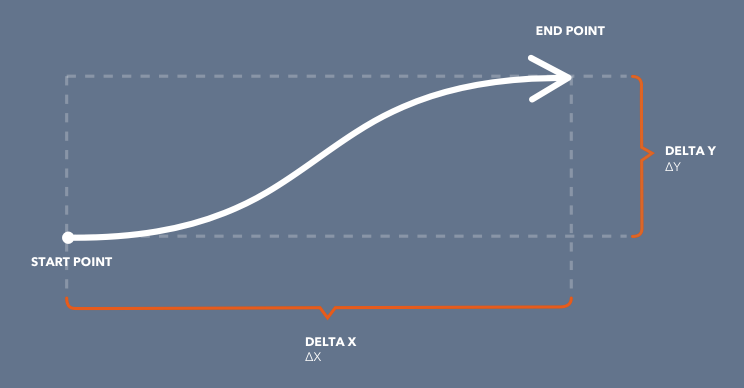Visual display of value differences (deltas), which we will need for further calculations

We can write a function to get all the points needed to draw the curve:

## Keep extreme values inline

Extreme values can be considered like maxima and minima on the curve function.

At Productboard, we have a very special requirement for not moving with these extreme values, because the space between the individual cards in the roadmap is limited, and we want the turn to always be the same length, no matter how long the curve. To do this, we must customize coordinates of the Control points dynamically according to the current height and width of the curve.

This part is a bit tricky, and it doesn’t have to be a use case for everyone, so don’t hesitate to skip over it if it doesn’t pertain to your situation.

The Bezier curve does not guarantee the same position of the extreme value when we drag with another point. See the following animation: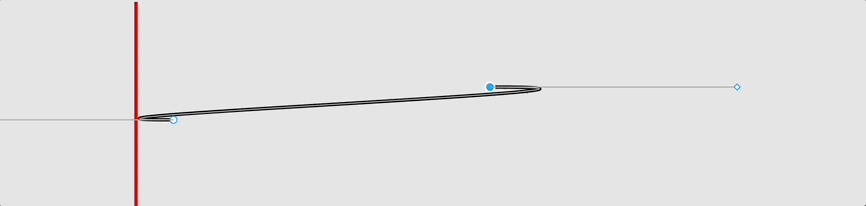In this animation, you can see how the extreme (leftmost edge of the curve) of the left point changes depending on where the right point of the curve is located. This is a characteristic of the Bezier curve that must be taken into account.

In our case, we don’t want to move with the extreme value position at all. This means that whether we have a long or short curve, the distance between the extreme and the point (the Start or End point) should always be similar.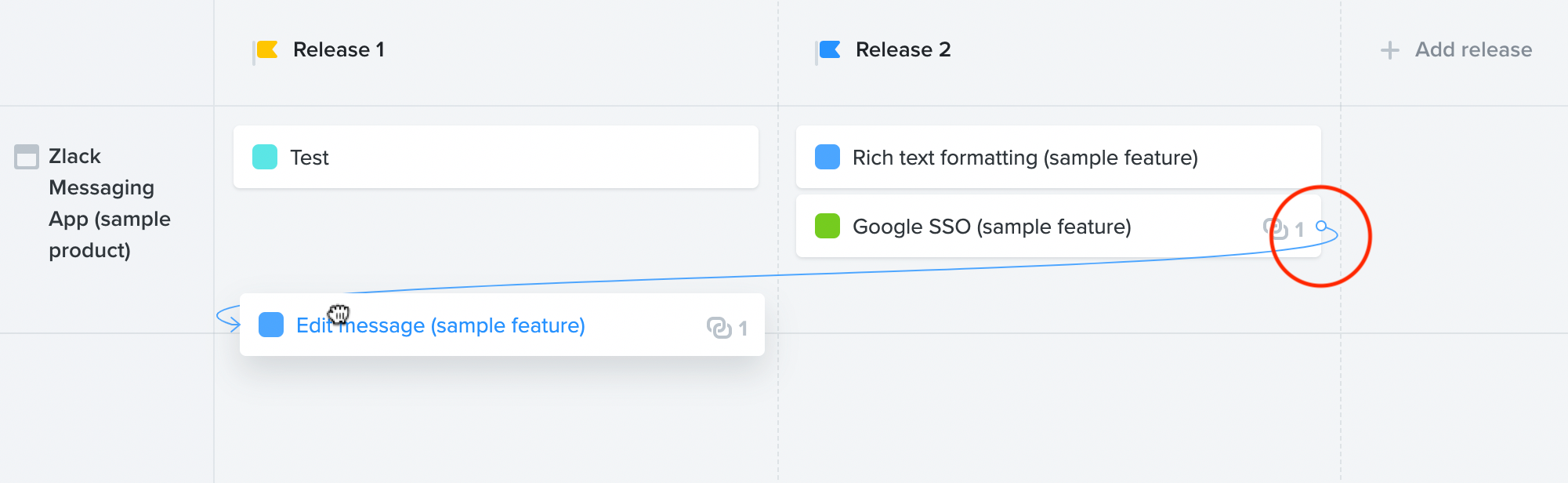In our case, the extreme should never cross the vertical light gray line, which is also inside the red circle

As can be seen from the pictures, the distance between the extreme value itself and the point with the small white dot is a similar length, although the classic Bezier curve does not behave in this way. So how did we do it?

Through observation, trial, and error, we tried to find a suitable formula for the correct shift of control points so that the extremes are always in the same position. We came up with the following formula:

Now let’s try to draw the following points:

The result looks pretty good: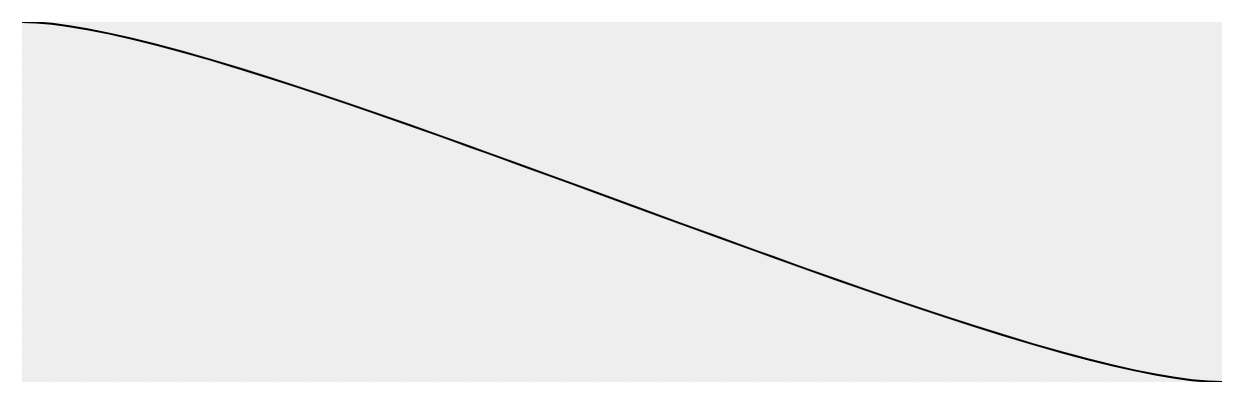Applying the Bezier curve to our implementation

## Enlargement of the bounding box

When we change the coordinates of the arrow (so that the X coordinate of the Start point is greater than X coordinate of the End point), we see that something is wrong: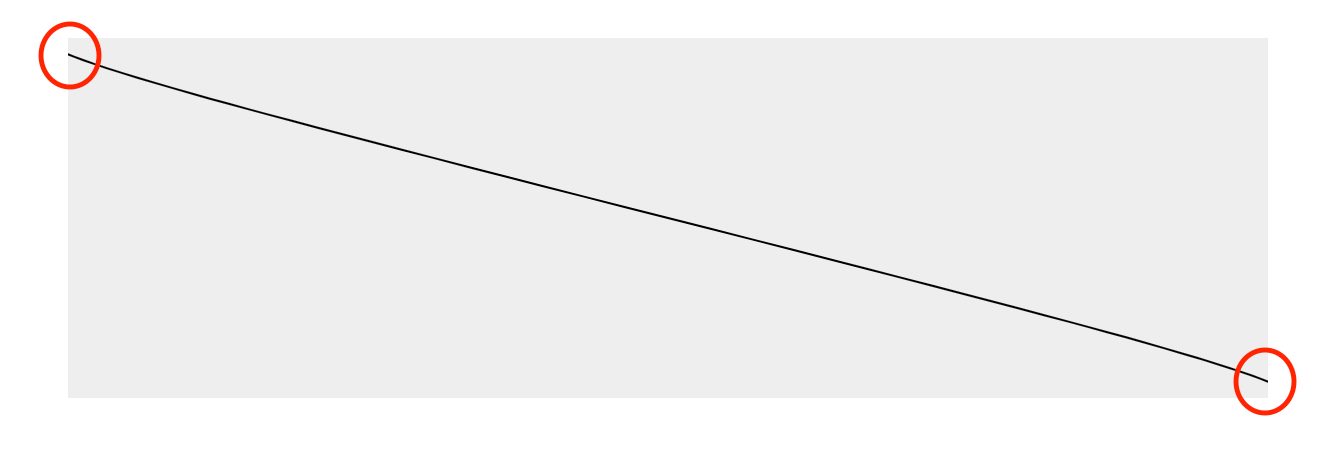The case when the control points are outside the bounding box and the whole bend of the curve is not drawn correctly

The problem is in the incorrect calculation of the bounding box (the gray rectangle in our case). Our improvement canvas does not take into account cases where the Control point of the curve is not drawn inside the bounding box.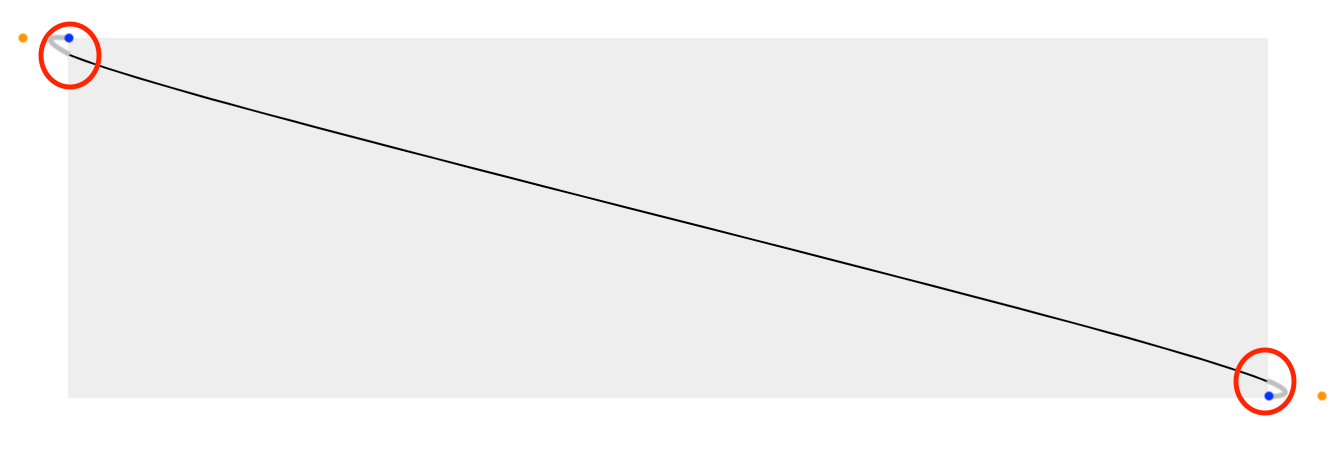The location of the Control points is marked in orange, the Start and End points are blue, and the whole color curve is marked in gray if we do not solve the bounding box.

Simply put, the bounding box needs to be enlarged.

The bounding box should be large enough to fit all the points that the curve contains. In addition, it is necessary to add the thickness of the line or the arrowhead, etc., if we do not want something to be cut. In the previous case, the bounding box should look something like this: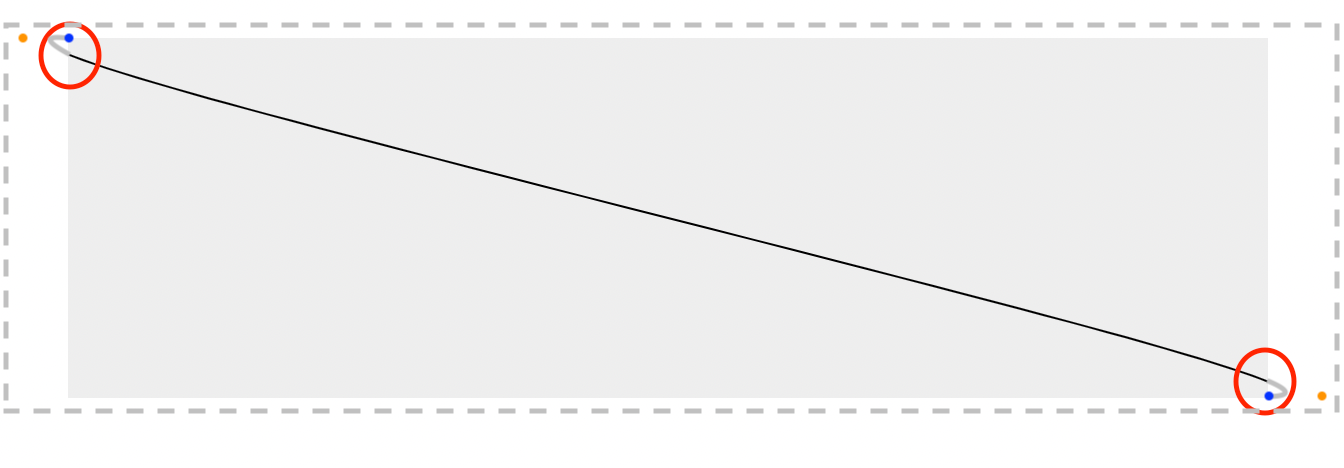The gray dashed line shows the bounding box that we need to calculate

For this reason, it is necessary to wrap calculation points into another function that adds the buffer to the size of the bounding box:

This gives us information about the size of the vertical and horizontal buffers, which need to be added to the size of the canvas. At the same time, it is necessary to move the canvas by half of this value to center it. We will do this using the following code:

After this adjustment, the bounding box is now the correct size: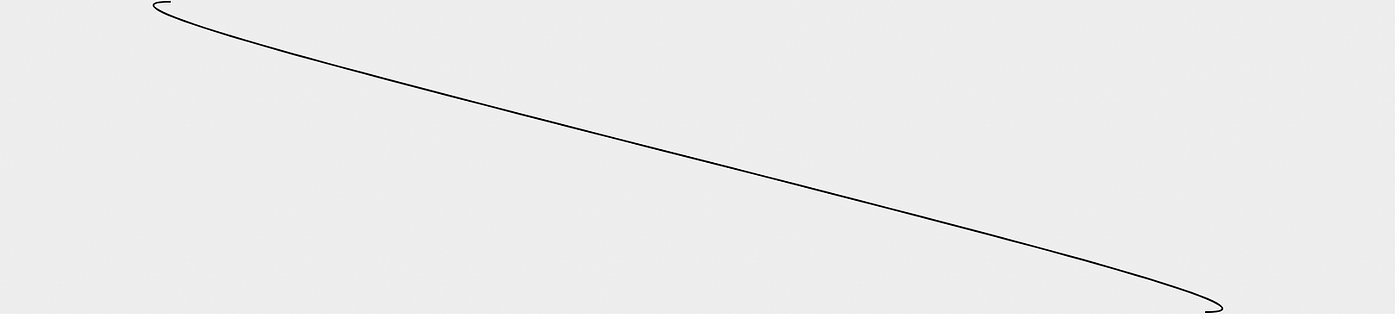Rendered arrow with extended canvas size to fit all the control points

## Follow-up

In the second part, we took an in-depth look at the representation of Bezier curves, calculating Control points, and customizing bounding box size.

In the LAST PART of this series, we will put in the final touches: We will draw an arrowhead, hook event listeners for interaction with the arrow component, and fix the last edge cases.

The whole implementation of SVG arrows in React is available on CodeSandbox or Github.

Interested in joining our growing team? Well, we’re hiring across the board! Check out our careers page for the latest vacancies.